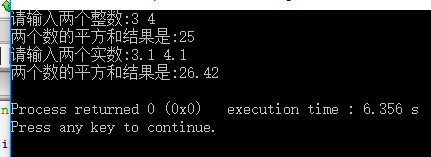# 函数的使用

1.为什么要用函数？
2.为什么要用函数重载？
3.什么是值传递？
4.什么是地址传递？
5.如何编写递归函数？

## 四.解决问题

### 2.为什么要用函数重载？

(a)函数重载是指两个以上的函数，具有相同的函数名，但是形参的个数或者类型不同，编译器根据实参和形参的类型及个数的自动匹配，自动确定调用哪一个函数，这就是函数的重载。(教材84页)
（b)注意：
1.不要将不同功能的函数定义为重载函数
2.重载函数的形参必须不同：个数不同或者类型不同

#include <iostream>
using namespace std;

int sum(int a,int b)
{

return a*a+b*b;
}
double sum(double a,double b)
{
return a*a+b*b;
}

int main()
{
int m,n;
cout<<"请输入两个整数:";
cin>>m>>n;
cout<<"两个数的平方和结果是:"<<sum(m,n)<<endl;

double x,y;
cout<<"请输入两个实数:";
cin>>x>>y;
cout<<"两个数的平方和结果是:"<<sum(x,y)<<endl;

return 0;
}### 3.什么是值传递？

#include <iostream>
using namespace std;

void swap(int a,int b){
int t=a;
a=b;
b=t;
}

int main()
{
int x=5,y=10;
cout<<"x="<<x<<"   y="<<y<<endl;
swap(x,y);
cout<<"x="<<x<<"   y="<<y<<endl;
return 0;
}



### 4.什么是地址传递

#include<iostream>
using namespace std;
int swap(int *a, int *b)
{
int t;
t = *a;
*a = *b;
*b = t;
return 0;
}
int main()
{
int x=5,y=10;
cout<<"x="<<x<<"   y="<<y<<endl;
swap(x,y);
cout<<"x="<<x<<"   y="<<y<<endl;
return 0;
}


5.如何编写递归函数？

#include<iostream>
using namespace std;

unsigned fac(unsigned n)
{
unsigned f;
if(n==0)
f=1;//递归终止条件
else
f=fac(n-1)*n;//应用递归函数
return f;
}
int main()
{
unsigned n;
cout<<"Enter a postitve interger:";
cin>>n;
unsigned y=fac(n);
cout<<n<<"!="<<y<<endl;
return 0;
}


void hanoi(int n,char src,char medium,char dest)//src为原始，medium为中转，dest为目标
{
if(n==1)
move(src,dest);
else{
hanoi(n-1,src,dest,medium);//对应的也分别是原始，中转和目标
move(src,dest);
hanoi(n-1,medium,src,dest);
}
}


posted on 2019-09-16 00:25  咬一口云朵软绵绵  阅读(239)  评论(0编辑  收藏  举报